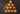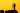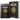# How to groupBy in JavaScript

by Robin Wieruch

The groupBy function is one of the functions why people use Lodash in their JavaScript code base. Here I want to give you a brief example on how to implement groupBy in vanilla JavaScript without Lodash by just using JavaScript's reduce method.

Let's say we have the following and we want to group them by property (here color) to get the following output:

```const users = [  { name: 'Jim', color: 'blue' },  { name: 'Sam', color: 'blue' },  { name: 'Eddie', color: 'green' },];
const usersByColor = // TODO: implement groupBy
console.log(usersByColor);
// {//   blue: [{//     { name: 'Jim', color: 'blue' },//     { name: 'Sam', color: 'blue' },//   }],//   green: [{ name: 'Eddie', color: 'green' }]// }```

We can use JavaScript's reduce method on an array to iterate over every item:

```const usersByColor = users.reduce((acc, value) => {  // TODO: implement groupBy
return acc;}, {});```

We start with an empty object as our accumulator (here `acc`) for this reduce's . For every iteration of the function, we return the changed (here still unchanged) accumulator. Let's implement groupBy:

```const usersByColor = users.reduce((acc, value) => {  if (!acc[value.color]) {    acc[value.color] = [];  }
// TODO: implement grouping
return acc;}, {});```

If the accumulator has no array initialized for the currently iterated value's color, we create an empty array for it allocated in the object whereas the color is the key. Afterward, we can assume that there is an array for the color and just push the value to it:

```const usersByColor = users.reduce((acc, value) => {  if (!acc[value.color]) {    acc[value.color] = [];  }
acc[value.color].push(value);
return acc;}, {});```

The groupBy in JavaScript is done. Here again with comments for both steps:

```const usersByColor = users.reduce((acc, value) => {  // Group initialization  if (!acc[value.color]) {    acc[value.color] = [];  }
// Grouping  acc[value.color].push(value);
return acc;}, {});```

Essentially we start with an empty object and for every iterated value, we negotiate whether we need to allocate a new array based on the property (here color) in this object. Afterward, we push the value to the (new) array.## How to countBy in JavaScript

The countBy function is one of the functions why people use Lodash in their JavaScript code base. Here I want to give you a brief example on how to implement countBy in vanilla JavaScript without…## Deep Dive into JavaScript's Array Map Method

The Map Function is one of the many Methods existing on the JavaScript Array prototype . If you want to do a deep dive on prototypical inheritance, here's a great read by Kyle Simpson on how…